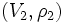# Direct sum of linear representations

This article gives a basic definition in the following area: linear representation theory
View other basic definitions in linear representation theory |View terms related to linear representation theory |View facts related to linear representation theory

## Definition

### Definition in terms of linear representation as a homomorphism

Suppose$(V_1,\rho_1)$ and$(V_2,\rho_2)$ are two linear representations of a group$G$ over a field$k$. In other words,$V_1, V_2$ are vector spaces over$k$ and$\rho_1:G \to Gl(V_1)$ and$\rho_2:G \to GL(V_2)$ are homomorphisms. Then, the direct sum is given as follows:

• The vector space is$V_1 \oplus V_2$
• Let$\alpha:GL(V_1) \times GL(V_2) \to GL(V_1 \oplus V_2)$ be the natural map obtained by coordinate-wise action. Then, the homomorphism from$G$ to$GL(V_1 \oplus V_2)$ is given by$\alpha \circ (\rho_1 \times \rho_2)$. In other words, the map is:$g \mapsto \alpha(\rho_1(g), \rho_2(g))$

In matrix terms, it$V_1$ and$V_2$ are finite-dimensional and are given a basis, we can think of$\rho_1,\rho_2$ as matrix-valued. Then the map is given as:$g \mapsto \begin{pmatrix} \rho_1(g) & 0 \\ 0 & \rho_2(g)\end{pmatrix}$

An analogous definition holds for infinite direct sums. Here,$\alpha$ is replaced by a map from the direct product of the$GL(V_i)$s to$GL(\bigoplus V_i)$.

### Definition in terms of linear representation as a group action

Suppose$G$ has two linear representations over a field$k$: one on a vector space$V_1$ and the other on the vector space$V_2$. Then, the direct sum representation on$V_1 \oplus V_2$ is given by making$G$ act coordinate-wise on the direct sum, i.e.:$g.(v_1,v_2) = (g.v_1,g.v_2)$

An analogous coordinate-wise definition holds for infinite direct sums.

### Definition in terms of linear representation as a module over the group ring

Suppose$G$ has two linear representations over a field$k$: modules$V_1$ and$V_2$ over the group ring$kG$. The direct sum of these representations is the direct sum of$V_1$ and$V_2$ as$kG$-modules.

Similarly, we can take an infinite direct sum of linear representations as the infinite direct sum of the corresponding modules.

## Facts

### Effect on character

Further information: sum of characters equals character of direct sum

The character of the direct sum of two finite-dimensional linear representations is the sum of their characters. This is because under the map:$\alpha:GL(V_1) \times GL(V_2) \to GL(V_1 \oplus V_2)$

The trace of$\alpha(a,b)$ is the sum of the traces of$a$ and$b$.

### Effect on determinant

The determinantal representation of the direct sum of two finite-dimensional linear representations is the product of their determinantal representations. That's because, the determinant of$\alpha(a,b)$ is the product of the determinants of$a$ and$b$.

### Indecomposable, irreducible, and completely reducible linear representations

A linear representation that cannot be expressed as a direct sum of two linear representations on nonzero vector spaces, is termed indecomposable.

A stronger notion than indecomposability is the notion of an irreducible linear representation: a linear representation that has no proper nonzero invariant subspace. Maschke's lemma says that in the non-modular case for a finite group (i.e., the case where the characteristic of the field does not divide the order of the group), every indecomposable representation is irreducible.

A representation that can be expressed as a direct sum (possibly infinite) of irreducible linear representations is termed a completely reducible linear representation. It turns out that if every indecomposable linear representation is irreducible, then every representation is completely reducible.